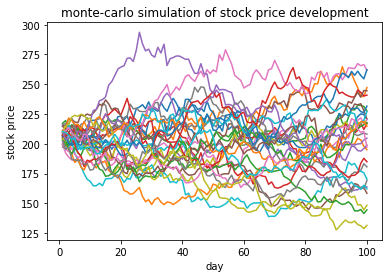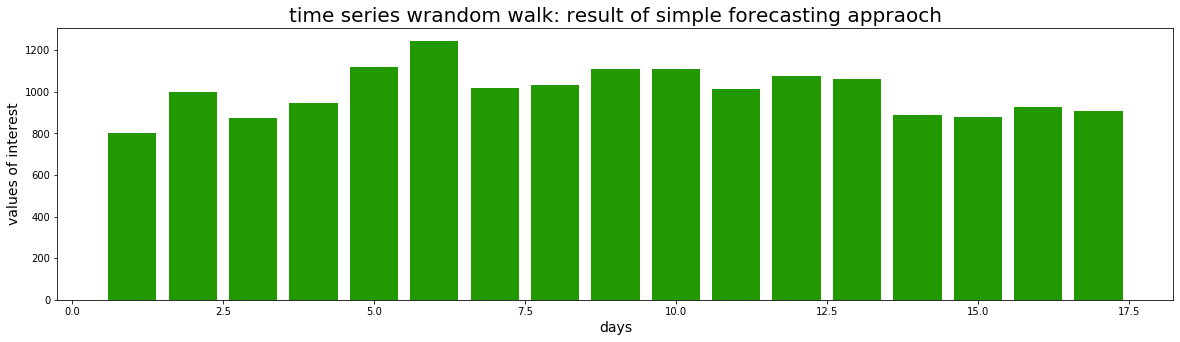# Monte-carlo simulation [EN]

## Simulation technique for SCM: Selection criteria for SCM analystsSimulation is becoming increasingly popular for factory planning, process design, supply chain optimization and supply chain management. In this article I provide a basic overview and introduction of relevant simulation techniques. It is important to point out that when choosing […]

## Monte-carlo simulation of stock prices (using Python)In one of my posts I have introduced the concept of random walk forecasting, using Python for implementation. In this post I want to conduct a monte carlo simulation of stock price random walks. For this I will use a […]

## Implementing a random walk forecast in PythonIn previous posts I introduced very simple (and naive) forecasting methods, namely CAGR-based forecasting and simple moving average forecasting. I implemented such forecasting methods in R and demonstrated basic use cases. In this post I want to introduce another simple […]